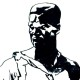n o f u t u r e b u t t h e w i n d s t i l l b l o w s t h r o u g h t h e t r e e s p l ay s w i t h t h e l e a v e s a n d m o v e s w h e r e i t w i l l a n d s o m e o f u s a r e l i k e t h a t s t i l l a n d w o n ‘ t b e t o l d s o l d o l d o r p i t t e d a g a i n s t a n o t h e r ‘ s b r e a t h . .
1.slpmartin says: